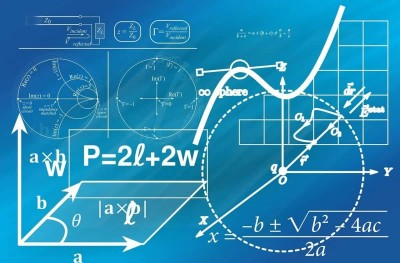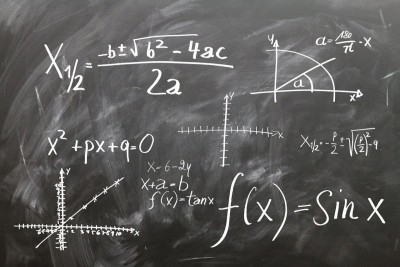# Math Courses In High School (What Math Classes To Take)

If you are getting ready to start high school or wondering what courses to take, it helps to have an idea of what to expect.  The math courses you take in high school depend on your previous course work in earlier grade levels.

So, what math courses do you take in high school?  High school math courses include Algebra 1 for 9th graders (freshmen), Geometry for 10th graders (sophomores), Algebra 2 for 11th graders (juniors), and Pre-calculus for 12th graders (seniors). Advanced students may take other math electives, such as AP Calculus, AP Statistics, or IB math courses.

Of course, you might also be able to take summer courses or online courses to get advanced standing in math courses or to qualify for AP courses.

In this article, we’ll talk about the math courses you take in high school.  We’ll also take a brief look at the list of topics covered in each of these courses.

Let’s get started.

## Math Courses In High School

In high school, most students will take the following order of math classes in high school:

• 9th grade: Algebra 1 (freshman year)
• 10th grade: Geometry (sophomore year)
• 11th grade: Algebra 2 (junior year)
• 12th grade: Pre-calculus (senior year)

If you have advanced standing due to extra courses taken (online classes, dual enrollment courses at a college, etc.), then you may skip ahead or take electives like AP Statistics.

### What Math Do 9th Graders Take? (Math Courses For High School Freshmen)

Most 9th graders in the U.S. will take Algebra I.  An Algebra 1 course includes topics such as:

How Much Time Does it Take to Learn...
How Much Time Does it Take to Learn Advanced Excel
• Linear equations
• Linear functions
• Inequalities
• Systems of linear equations
• Systems of inequalities
• Exponent rules (powers)
• Roots and radicals

A more advanced 9th grader may take Algebra 2 or Geometry.  Of course, this depends on the student’s math background from 8th grade.

If a 9th grader has already taken the algebra prerequisites, an elective course such as probability or statistics is also possible.

An SAT or ACT math prep course might also be a good idea in 9th grade.  This is especially true if you want to qualify for competitive scholarships and improve your chances of winning.

If you are a 9th grader and you want to compete in mathematics at the national level, consider taking a course to prepare for the AMC 10.  This math exam elementary algebra, geometry, number theory, and probability (there is no advanced algebra, geometry, or trigonometry).

You can take the AMC 10 if on the day of the contest you are:

• In 10th grade or below
• Age 17.5 years or below

### What Math Do 10th Graders Take? (Math Courses For High School Sophomores)

Most 10th graders in the U.S. will take Geometry.  A Geometry course includes topics such as:

• Congruence
• Similarity
• Symmetry
• Right Triangles
• Trigonometry
• Circles
• Conic Section Equations
• Area
• VolumeA high school geometry course will include topics on triangles, circles, area, and volume.

However, in some schools, this course has been phased out in favor of algebra courses that teach some geometry along the way.  In that case, you might take Algebra 2 (advanced algebra) in 10th grade.

A more advanced 10th grader may also take Algebra 2 or even a pre-calculus course.  Of course, this depends on the student’s math background from 9th grade.

If a 10th grader has already taken the algebra prerequisites, an elective course such as probability or statistics is also possible.

An SAT or ACT math prep course is a good idea in 10th grade.  This is especially true if you want to qualify for competitive scholarships and improve your chances of winning.

If you are a 10th grader and you want to compete in mathematics at the national level, consider taking a course to prepare for the AMC 10.  This math exam elementary algebra, geometry, number theory, and probability (there is no advanced algebra, geometry, or trigonometry).

You can take the AMC 10 if on the day of the contest you are:

• In 10th grade or below
• Age 17.5 years or below

### What Math Do 11th Graders Take? (Math Courses For High School Juniors)

Most 11th graders in the U.S. will take Algebra 2.  An Algebra 2 course includes topics such as:

• Linear equations
• Systems of linear equations
• Functions (absolute value, piecewise, step, inverse)
• Inequalities
• Systems of inequalities
• Polynomials
• Power Functions
• Exponents
• Logarithms
• Rational Equations
• Complex (Imaginary) NumbersA high school Algebra 2 course may include more advanced treatment of quadratics, along with complex (imaginary) numbers and logarithms.

A more advanced 11th grader may take a pre-calculus or even a calculus course.  Of course, this depends on the student’s math background from 10th grade.

If an 11th grader has already taken the algebra prerequisites, an elective course such as probability or statistics is also possible.  These courses could be AP or IB, which are geared towards preparing for exams that grant college credit.

Taking a dual enrollment course at a nearby college (or an online course) gives you the chance to earn both high school and college credits at the same time.

An SAT or ACT math prep course is also a good idea in 11th grade.  This is especially true if you want to qualify for competitive scholarships and improve your chances of winning.

If you are an 11th grader and you want to compete in mathematics at the national level, consider taking a course to prepare for the AMC 12.  This math exam covers all of the topics on the AMC 10 plus trigonometry, advanced algebra, and advanced geometry.

You can take the AMC 12 if on the day of the contest you are:

• In 12th grade or below
• Age 19.5 years or below

### What Math Do 12th Graders Take? (Math Courses For High School Seniors)

Most 12th graders in the U.S. will take Pre-calculus.  A Pre-calculus includes topics such as:

• Functions
• Function composition
• Inverse functions
• Polynomials
• Rational functions
• Trigonometric functions
• Trigonometric identities
• LimitsA high school Pre-calculus course will prepare you for calculus in college.

A more advanced 12th grader may take a calculus course.  This depends on the student’s math background from 11th grade.

If a 12th grader has already taken the prerequisites, an elective course such as AP Calculus or AP Statistics is also possible.  These courses are geared towards preparing for exams that grant college credit.

Taking a dual enrollment course at a nearby college (or an online course) gives you the chance to earn both high school and college credits at the same time.

An SAT or ACT math prep course is also a good idea in 12th grade (in the fall – spring will be too late to take the SAT or ACT and apply to college for spring admission).  This is especially true if you want to qualify for competitive scholarships and improve your chances of winning.

If you are a 12th grader and you want to compete in mathematics at the national level, consider taking a course to prepare for the AMC 12.  This math exam covers all of the topics on the AMC 10 plus trigonometry, advanced algebra, and advanced geometry.

You can take the AMC 12 if on the day of the contest you are:

• In 12th grade or below
• Age 19.5 years or below

## Conclusion

Now you know which math courses you take in high school and when you can expect to take them.  Remember that there is some variability depending on the school and your background from earlier grade levels.

If you are interested in online schools in Massachusetts, check out my article here.

You might also consider joining a local (or virtual) math circle to hone your mathematical abilities – learn more in my article here.

If you would like to hire a math tutor, check out this article first.

I hope you found this article helpful.  If so, please share it with someone who can use the information.

~Jonathon